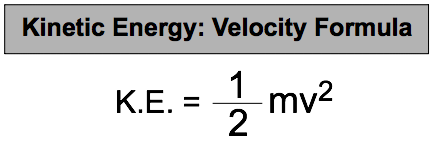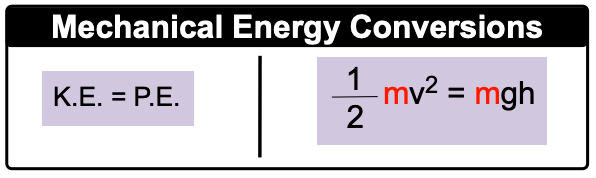Clutch Prep is now a part of Pearson
Ch.6 - Thermochemistry WorksheetSee all chapters

# Kinetic & Potential Energy

See all sections
Sections
Nature of Energy
Kinetic & Potential Energy
First Law of Thermodynamics
Internal Energy
Endothermic & Exothermic Reactions
Heat Capacity
Constant-Pressure Calorimetry
Constant-Volume Calorimetry
Thermal Equilibrium
Thermochemical Equations
Formation Equations
Enthalpy of Formation
Hess's Law
Enthalpy (IGNORE)

Mechanical Energy is the energy associated with an object's velocity (Kinetic Energy) and its position (Potential Energy).

###### Kinetic Energy & Potential Energy

Concept #1: Kinetic & Potential Energy

The kinetic energy of a gas molecule is connected to its mass in kilograms and velocity in meters per second.The potential energy of a gas molecule is connected to its mass, acceleration due to gravity and height above the ground.Example #1: Calculate the kinetic energy (in kJ) of an electron (m = 9.11 x 10-31 kg) moving at 1.59 x 1020 m/s.

Practice: A radioactive particle weighing 7.20 x 103 ng is found 110 m above the earth’s surface. What is its potential energy?

Concept #2: Energy InterconversionsExample #2: A neutron weighing 1.67 x 10-27 kg is shot from a laser projector that is mounted 120.0 meters above the ground. What is its speed when it hits the ground?